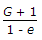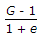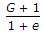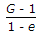# Civil Engineering - UPSC Civil Service Exam Questions

26.

Which one of the following pairs relating to flumes carrying open channel flow is correctly matched ?

 A. N-modular flume....Flow is unaffected by drowning B. Venturi flume....Standing wave forms at the throat C. Venturi flume....Flow at the throat is at less than critical velocity D. Standing wave flume....Hump is not provided at the throat

Explanation:

No answer description available for this question. Let us discuss.

27.

A cylindrical vessel with a constant plane area of 1 m2 is rotated about its vertical axis such that the liquid inside the vessel is about to spill. If the height of the vessel is 2 m and the height of the paraboloid at 1 m, then the volume (in m3) of the liquid in the vessel will be

 A. 2 B. 1.5 C. 1 D. 0.5

Explanation:

No answer description available for this question. Let us discuss.

28.

Critical hydraulic gradient ic is given by (where, G = specific gravity of solids e = void ratio)

 A.B.C.D.Explanation:

No answer description available for this question. Let us discuss.

29.

Consider the following statements with reference to oxidation ponds :
1. They require high initial investments
2. They have low operational cost
3. They are also called stabilization ponds.
Which of the statements given above are correct ?

 A. 1 and 2 B. 2 and 3 C. 1 and 3 D. 1, 2 and 3

Explanation:

No answer description available for this question. Let us discuss.

30.

The length, coefficient of thermal expansion and Young's modulus of bar 'A' are twice that of bar 'B'. If the temperature of both bars is increased by the same amount while preventing any expansion, then the ratio of stress developed in bar A to that in bar B will be

 A. 2 B. 4 C. 8 D. 16